# Omega being less than one in a flat Universe?

TheTraceur
Hi everyone! I'm not very good at math as I'm in high school, and so probably did something wrong in this, but I did some calculations and am now wondering if a flat Universe requires Ω0 ≠ 1?

I started with the Friedmann equation,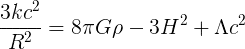If you divide by 3 and take the inverse of both sides, you get,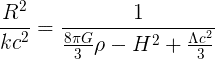Since,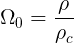Where ρc is the critical density, we can re-write the equation in terms of Omega (I also multiplied both sides by c2),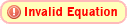Here it to me looks a bit odd, because here Omega being one does not correspond to a Universe with infinite radius of curvature, since you still have to cosmological constant, which is positive. Here it seems that if you want to assume that k = 0, and so have a flat Universe, you will need to have (since if R2/k, where k = 0, is 'infinite', then the other side must also be 'infinite'):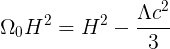We then get: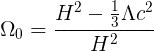And since the cosmological constant has been discovered to be positive, this would seem to mean that for a flat Universe (k = 0), Ω0 is not exactly equal to one, but in fact a tiny bit smaller. I'm pretty sure this is false, but I don't know where I've missed the fault in the derivation. May it be that the cosmological constant is assumed to be zero in the definition of critical density? Because if you don't assume a zero value for the cosmological constant in the definition of critical density, the cosmological constant dissapears in the denominator, and so Omega being one corresponds to a flat Universe.

Last edited:

Staff Emeritus
Gold Member
Welcome to PF, TheTraceur!

Hi everyone! I'm not very good at math as I'm in high school, and so probably did something wrong in this, but I did some calculations and am now wondering if a flat Universe requires Ω0 ≠ 1?

I started with the Friedmann equation,If you divide by 3 and take the inverse of both sides, you get,Since,Where ρc is the critical density, we can re-write the equation in terms of Omega (I also multiplied both sides by c2),Here it to me looks a bit odd, because here Omega being one does not correspond to a Universe with infinite radius of curvature, since you still have to cosmological constant, which is positive. Here it seems that if you want to assume that k = 0, and so have a flat Universe, you will need to have (since if R2/k, where k = 0, is 'infinite', then the other side must also be 'infinite'):

The thing you're missing here is that the ρ in your equation is the density of matter, ρm. So when you compute Ω0 = ρmcr, you're only computing Ωm, the density parameter for matter. This is less than 1: in the standard concordance model, we have Ωm = 0.27 and Ωde = 0.73, where Ωde (also called ##\Omega_\Lambda##) is the density parameter for dark energy. As you know, observations show that the total matter density is far less than the critical density, and it is the dark energy that makes up the difference, bringing the universe very close to, if not exactly, critical (flat).

So, how can your equation be reworked to include Ωde? Well, for the simplest type of dark energy, which is just represented by a cosmological constant term in the Friedmann equations, it turns out that the dark energy density ρde is constant with time, and is related to the cosmological constant by: ##\Lambda = 8\pi G \rho_{\textrm{de}}##

Substituting this into your equation above, we get (note, I'll just take c = 1 for convenience):$$H^2 = \frac{8\pi G}{3}\rho_m + \frac{8\pi G}{3}\rho_{\textrm{de}} - \frac{k}{R^2}$$Divide both sides of the equation by H2:$$1 = \frac{8 \pi G}{3H^2}\rho_m + \frac{8 \pi G}{3H^2}\rho_{\textrm{de}} - \frac{k}{R^2 H^2}$$Now, the important thing to understand in this equation is that a lot of these things are functions of time, i.e. their values change with cosmic time, t (the time since the Big Bang, according to observers who are co-moving with the expansion). The Hubble parameter, H(t) is a function of time. So is the matter density ρm(t). So is the scale factor R(t). I am assuming that you are using R to denote the scale factor. I usually use 'a', but that's okay. So, let's evaluate this equation NOW i.e. at t = t0, where t0 is the present age of the universe (some 13.7 billion years). It turns out that the present value of the Hubble parameter, H(t0), is just equal to the Hubble constant, H0. The present value of the scale factor, R(t0) = 1. I'll call the present value of the matter density ρm,0. So at t = t0 the equation becomes:$$1 = \frac{8 \pi G}{3H_0^2}\rho_{m,0} + \frac{8 \pi G}{3H_0^2}\rho_{\textrm{de}} - \frac{k}{H_0^2}$$ By now, you probably recognize that the factors in front of the ρ's are just equal to the reciprocal of the critical density: ##\rho_{\textrm{cr}} = 3H_0^2 / (8\pi G)##. So the equation becomes:$$1 =\frac{\rho_{m,0}}{\rho_{\textrm{cr}}} + \frac{\rho_{\textrm{de}}}{\rho_{\textrm{cr}}} - \frac{k}{H_0^2}$$By the definition of the Ω's, this is just equal to:$$1 = \Omega_m + \Omega_{\textrm{de}} - \frac{k}{H_0^2}$$OR$$\frac{k}{H_0^2} = (\Omega_m + \Omega_{\textrm{de}}) - 1$$$$\frac{k}{H_0^2} = \Omega_{\textrm{total}} - 1$$So if Ωtotal (including contributions from both matter and dark energy) differs from 1, then the curvature k is non-zero. Some people even go so far as to define a "curvature" density parameter Ωk = -k/H02, so that 1 = Ωm + Ωde + Ωk.

Last edited: